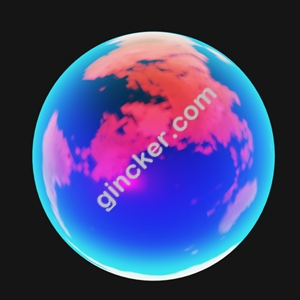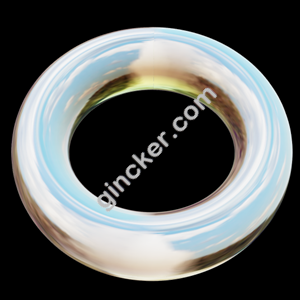### Free Ginckers

#### Bouligand Cushion

Template: Graphics/3d-parametric-shape

Discription: This template creates a parametric Bouligand cushion shape with a set of parametric equations.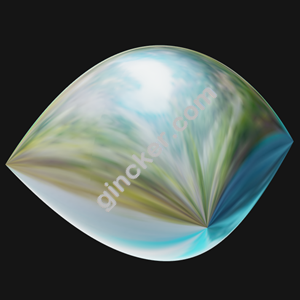#### Circled Helicoid

Template: Graphics/3d-parametric-shape

Discription: The circled helicoid can be generated by the helical movement of a circle. This surface is created by extending a sphere along a diameter and then twisting.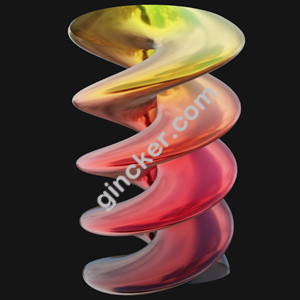#### Cosine-Sine Shape

Template: Graphics/3d-parametric-shape

Discription: This template creates a 3D surface with a set of parametric cosine-sine equations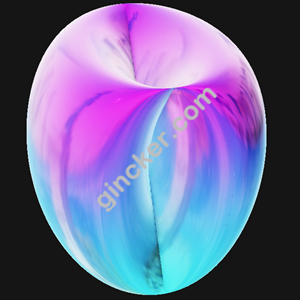#### Cross Gap

Template: Graphics/3d-parametric-shape

Discription: Cross-gap is a representation of the projective plane. It is like a shrinked torus where there is no middle hole and the side has been pinched together in such a way that the top cross to the bottom.#### Dini Shape

Template: Graphics/3d-parametric-shape

Discription: Dini shape is a surface with constant negative curvature that can be created by twisting a pseudosphere. It is named after Ulisse Dini.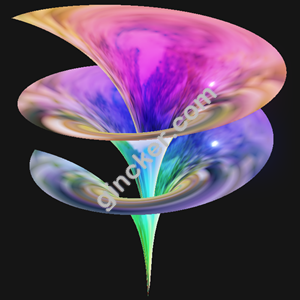#### Drop Shape

Template: Graphics/3d-parametric-shape

Discription: This template creates a drop shape with a set of parametric equations.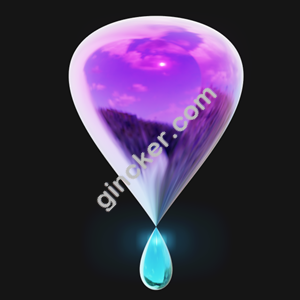#### Elliptic Cone

Template: Graphics/3d-parametric-shape

Discription: An elliptic cone is a type of quadric surfaces. A quadric surface is a generalization of conic sections (ellipses, parabolas, and hyperbolas).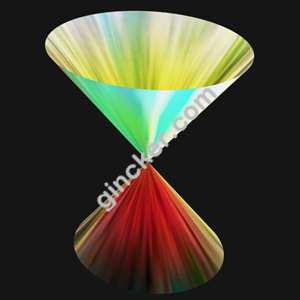#### Elliptic Cyclide

Template: Graphics/3d-parametric-shape

Discription: Elliptic cyclide is a type of Dupin cyclides. Dupin cyclide is any geometric inversion of a standard torus, cylinder, or double cone. Dupin cyclides are natural objects in Lie sphere geometry.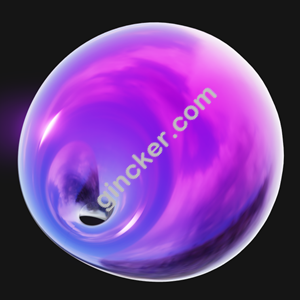#### Globoid Shape

Template: Graphics/3d-parametric-shape

Discription: The Globoid surface can be generated by rotation of an arc of the circle about the z-axis lying at the plane of the arc.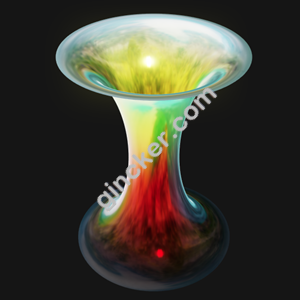#### Helicoid

Template: Graphics/3d-parametric-shape

Discription: A helicoid is a trace of a line. For any point on the surface, there is a line on the surface passing through it. Helicoids are shaped like screws and can be described be a set of parametric equations.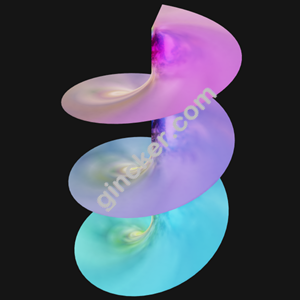#### Klein Bottle

Template: Graphics/3d-parametric-shape

Discription: A Klein bottle is an example of a non-orientable surface. It is a 2D manifold against which a system for determining a normal vector cannot be consistently defined. It is a one-side surface that, if traveled upon, could be followed back to the point of origin while flipping the traveler upside down.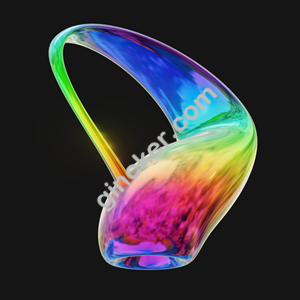#### Peaks

Template: Graphics/3d-parametric-shape

Discription: This template creates a simple 3D shape for a mathematical peaks function with two parametric variables u and v.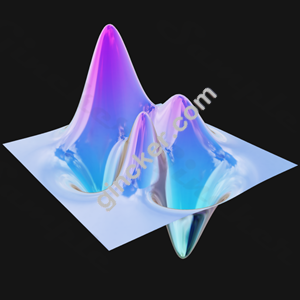#### Sinc

Template: Graphics/3d-parametric-shape

Discription: This template creates a simple 3D shape for a mathematical sinc function with two variables u and v.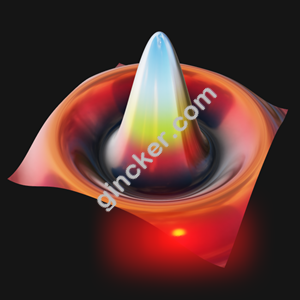#### SincXY

Template: Graphics/3d-parametric-shape

Discription: This template creates a simple 3D shape for a mathematical sincXY function with two parametric variables u and v.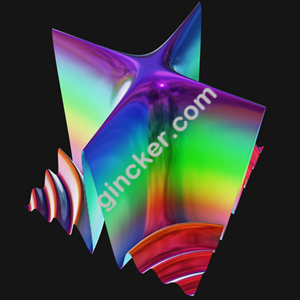#### Soucoupoid Shape

Template: Graphics/3d-parametric-shape

Discription: This template creates a parametric soucoupoid surface with a set of parametric equations.#### Sphere

Template: Graphics/3d-parametric-shape

Discription: This template creates a parametric sphere surface with a set of parametric equations.